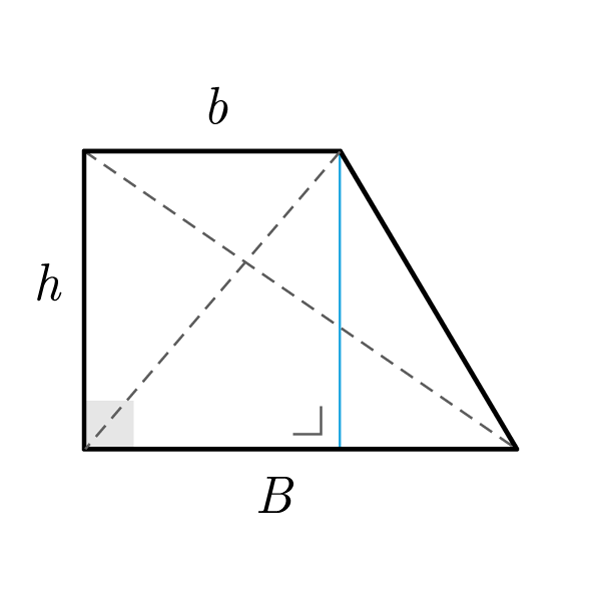# Right Trapezoid

 Longer Base $$B$$ Shorter Base $$b$$ Height $$h$$ Oblique Side $$S$$ Oblique side Projection $$p_{1}$$ Longer Diagonal $$d_{1}$$ Shorter Diagonal $$d_{2}$$
$$2p = B + b + S + h$$
Perimeter
$$A = \frac{\left(B + b \right) \times h}{2}$$
Area
$$B + b = \frac{2A}{h}$$
Sum of bases
$$h = \frac{2A}{B + b}$$
Height
$$p_{1} = B - b$$
Oblique side Projection
$$B - b = p_{1}$$
Difference of bases
$$B = b + p_{1}$$
$$b = B - p_{1}$$
Right Tr. delimited by height - oblique side
$$S = \sqrt{ {p_{1}}^2 + {h}^2 }$$
Side (Pythagoras' theorem)
$$p_{1} = \sqrt{ {S}^2 - {h}^2 }$$
Oblique side Projection
$$h = \sqrt{ {S}^2 - {p_{1}}^2 }$$
Height
Right Tr. delimited by height - longer diagonal
$$d_{1} = \sqrt{ {B}^2 + {h}^2 }$$
Longer Diagonal (Pythagoras' theorem)
$$B = \sqrt{ {d_{1}}^2 - {h}^2 }$$
Longer Base
$$h = \sqrt{ {d_{1}}^2 - {B}^2 }$$
Height
Right Tr. delimited by height - shorter diagonal
$$d_{2} = \sqrt{ {b}^2 + {h}^2 }$$
Shorter Diagonal (Pythagoras' theorem)
$$b = \sqrt{ {d_{2}}^2 - {h}^2 }$$
Shorter Base
$$h = \sqrt{ {d_{2}}^2 - {b}^2 }$$
Height

## Definition

A right trapezoid is a trapezoid with a right angle (90 degree).

## Properties

1. It has a right angle (90 degree)
2. All the  Generic Trapezoid formulas are valid## Right Trapezoid Formulas

Data Formula
Perimeter 2p = B + b + S + h
Area A = [(B + b) × h] / 2
Height h = (2 × A) / (B + b)
Oblique side Projection p1 = B - b
Sum of bases B + b = (2 × A) / h
Sum of bases B + b = 2p - 2 × S